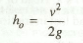# Loss of Head due to Friction in Pipe

How do we calculate Loss of Head due to Friction in Pipe? What is the major cause of loss of head or energy in a pipe?

According to Darcy's formula, the loss of head due to friction in the pipe (hf) is given by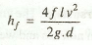where

f = Darcy's coefficient,
l = Length of pipe,
v = Mean velocity of liquid in pipe, and
d = Diameter of pipe.

The major loss of head or energy is due to friction. The minor loss of head includes the following cases:

(a) Loss of head due to sudden enlargement,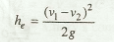(b) Loss of head due to sudden contraction,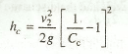where

Cc= Coefficient of contraction.

(c) Loss of head at the inlet of a pipe,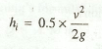(d) Loss of head at the outlet of a pipe,